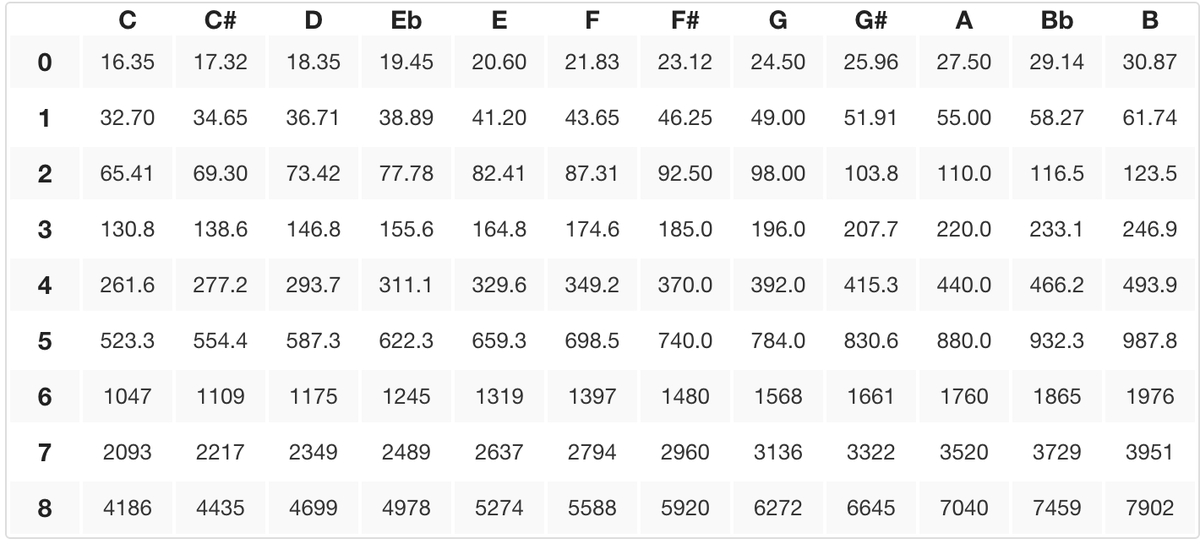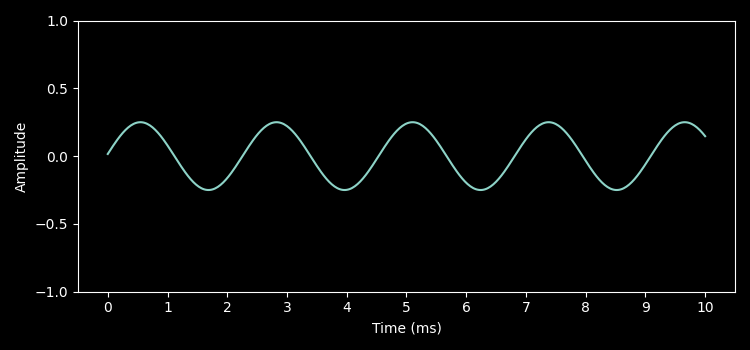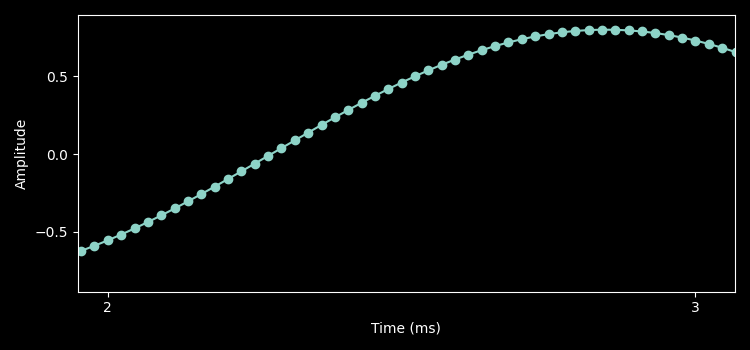# Playing a single note with SDL2

I have successfully avoided sound programming for all my programming life. Even when I tried making games, sound was always an afterthought. However, recently I got a new pair of headphones, and wondered: “what makes sound good?”, or “how much of the quality can be improved by hardware?”, and even “what is the structure of a sound file?”. If you’re looking for answers to all these questions — sorry, you are in the wrong place. I don’t have them (yet). But I can offer something equally fun: make your computer play a note by writing less than 100 lines of C code.

If you’re here just for the end result: here it is.

### Frequency, amplitude and sample rates

Before jumping into C code, let’s review some fundamentals.

In this exercise, we’ll see periodic signals, which are signals that repeat themselves every T amount of time. You have probably seen an image like this before:This is a sinusoid function for a A4 note (440Hz). An undulating curve that rises and falls, representing the sound wave.

Let’s break it down:

Each repetition of a periodic signal is called a cycle. A frequency (Hz) is the rate at which the signal completes a cycle (i.e. how often it oscillates). If there are 100 cycles per second, the frequency is 100Hz.

What we perceive as notes are sounds with specific frequencies. In the example above, the note A4 is when the cycle oscillations happen 440 time per second. A sine wave that oscillates 880 time per second is still the same note (A) but at a higher octave (A5).The table above shows the frequency value for each note. Changing our sine wave frequency from 440 to 523.3 would change the sound from an A4 to a C5. We are not getting into the music theory part of things in this post. If you’re interested in reading more about this, look up harmonics and pitch notation.

The amplitude is how tall the wave is. The higher the distance between its peaks, the louder we perceive the sound to be. Here’s a wave that is also an A4 (440Hz), but with a smaller amplitude:Playing this wave would result in the same but quieter sound than the wave above

To encode a A4 sine wave, we need to sample points in this signal and feed them to the sound card. The sinusoid function above has theoretically infinite points between 1 and 2 milliseconds. The challenge is: how often shall we sample the points to reproduce this sine wave with high enough fidelity? In signal processing, this is called sample rate. Sample rate is how many points are being sampled per second.

Zooming in between millisecond 2 and 3 in the graph above shows where the samples are considering 44100 samples per second:A high sample rate is necessary to avoid folding frequencies. This is when a frequency is so high that multiple cycles can occur between the sampling rate. When this happens, it’s impossible to distinguish high frequencies from their higher pitch counterparts. The Nyquist theorem states you need at least double the sample rate of the highest frequency you want to capture governs this. With 44100Hz, we should cover the highest frequency the human ear can hear.

A word of caution (!): Always test the sound initially at a low volume and gradually increase as needed. Unexpected loud noises could lead to permanent hearing damage.

### SDL Sound API

Now that we understand what frequencies are and why we need a sample rate, let’s focus on how to get this A4 note to play using code.

There are two ways of telling your sound card that a sound has to be played. You can queue samples, or you can register a callback to feed the audio data. In this example, I’m using the callback, because that looked simpler.

``````void callback(void *userdata, Uint8 *stream, int len) {
for (int i = 0; i < len; i++) {
stream[i] = /* ADD SAMPLE HERE */ ;
}
}
``````

SDL audio callback:

• `userdata`: provide custom data to the callback, this is useful if you want to scope user configurations (e.g. volume) to be passed on each callback.
• `stream`: a buffer, managed by SDL to be filled with sample points that will be fed to the sound card.
• `len`: the size of the buffer in bytes.
``````const int SAMPLE_RATE = 44100;
const int BUFFER_SIZE = 4096;

int main() {
if (SDL_Init(SDL_INIT_AUDIO | SDL_INIT_EVENTS) < 0) {
printf("Failed to initialize SDL: %s\n", SDL_GetError());
return 1;
}
SDL_AudioSpec spec = {
.format = AUDIO_F32,
.channels = 1,
.freq = SAMPLE_RATE,
.samples = BUFFER_SIZE,
.callback = callback,
};

if (SDL_OpenAudio(&spec, NULL) < 0) {
printf("Failed to open Audio Device: %s\n", SDL_GetError());
return 1;
}

SDL_PauseAudio(0);
while (true) {
SDL_Event e;
while (SDL_PollEvent(&e)) {
switch (e.type) {
case SDL_QUIT:
return 0;
}
}
}
return 0;
}
``````

SDL boilerplate program that will play the audio fed by the callback until `SDL_QUIT` is received

`SDL_AudioSpec.samples` specify how many frames the buffer shall have. I.e. this is how many samples need to be provided when the callback is called. This value is multiplied by the number of channels requested.

The `SDL_AudioSpec.format` is the primitive type used to fill the callback buffer. By default, this is an 8bit integer, but in this example I’m using a 32bit floating point. This means that the callback has to fill in `4096 * sizeof(float) * spec.channels` bytes in buffer.

Using a 32bit floating point provides a higher precision for audio samples compared to the default 8bit integer. As we’ll see in the following section, this makes it easier to work with the `sin` functions since it already returns floating points.

### Writing a sine wave function at 440Hz

The challenge now is to write a function that generates the correct number of samples given a frequency. At first, it sounded like a mystery to me, but you can answer that with some basic arithmetic.

• A sine function takes an angle of `π` radians to go from its highest point to its lowest, and consequently `2π` to complete a cycle.
• To produce an A4 note, our `x` value has to move `2π` 440 times in one second.
• With 44100 sample rate, every `44100/440` samples `x` has to move `2π`.

Given these facts, we have all we need to write a function that produces a `440Hz` sine wave function.

Here’s a simple generic code that can wrap the generation of sample points for any frequency:

``````typedef struct {
float current_step;
float step_size;
float volume;
} oscillator;

oscillator oscillate(float rate, float volume) {
oscillator o = {
.current_step = 0,
.volume = volume,
.step_size = (2 * M_PI) / rate,
};
return o;
}

float next(oscillator *os) {
os->current_step += os->step_size;
return sinf(os->current_step) * os->volume;
}

``````

Given a `rate` the `oscillator` struct will keep producing floating points along the sine wave

With that, one could instantiate an oscillator to keep generating points along the sine wave:

``````float A4_freq = (float)SAMPLE_RATE / 440.00f;
a4 = oscillate(A4_freq, 0.8f)

next(a4); // returns the next sample point in the A4 sine wave
``````

### Providing samples to the callback function

Now that we have the sine wave function, we need to use it in our callback. Remember, as mentioned above, we are using 32-bit float as a format. This means we have some work to do to make sure we write to the correct segment in the buffer provided.

``````void oscillator_callback(void *userdata, Uint8 *stream, int len) {
float *fstream = (float *)stream;
for (int i = 0; i < BUFFER_SIZE; i++) {
float v = next(A4_oscillator);
fstream[i] = v;
}
}
``````

Assume `A4_oscillator` is a global `oscillator` instance, similar to the one above.

You may notice that the provided buffer is a `Uint8*`, which I was ignoring until now. There are no specific callbacks for the different formats, therefore, we need to cast the buffer to `float*` in order to write our sine wave floating points into it. Incorrectly handling or casting the buffer can lead to distorted audio output or even crashes.

Since `len` is the number of bytes the buffer has, we can’t iterate from `i` to `len`. Instead, we use `BUFFER_SIZE` here which is the number 32-bit float frames that need to be filled.

Remember that if you’re using multiple channels, this number will vary. We are using a single channel for simplicity

### Results and references

The entire code can be found here. There are some additional things that weren’t mentioned in code, like outputting the samples for debugging and plotting reasons. There is a also a python script to use `matplotlib` to generate the graphs shown in this post.

If you run the code, you should hear the A4 in the terminal until the program is exited. If you compare to the 440Hz sine wave sound generated in an online tone generator you will notice that it’s the same.

Thanks for getting to the end of this post. If you’re interested in more things audio programming, I can recommend the following resources I’ve used:

• Think DSP (Digital Signal Processing) in Python is a free book by Allen B. Downey. This is a beginner friendly book that walks through signal processing using Python. The first two chapters in the book cover in more depth the contents of this blog post.
• If you like long video formats, this 3Blue1Brown video helped me expand my mental model to actually understand sound frequencies, waveforms and the Fourier Transform.
• Additionally, this video by Tsoding about noise generators is very good and covers a lot of content related to SDL2 audio API.

⇦ Back Home | ⇧ Top |

If you hated this post, and can't keep it to yourself, consider sending me an e-mail at fred.rbittencourt@gmail.com or complain with me at Twitter X @derfrb. I'm also occasionally responsive to positive comments.